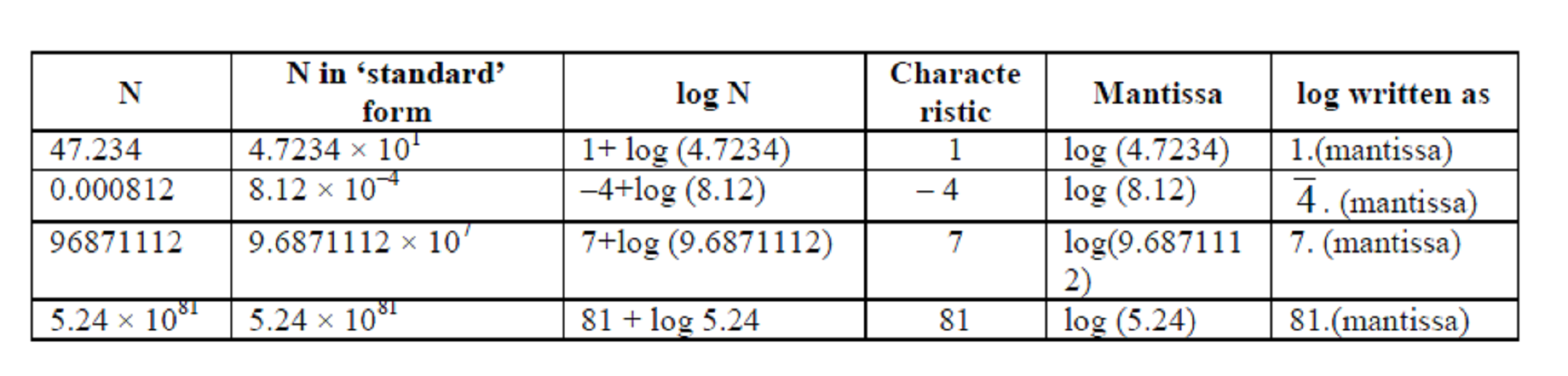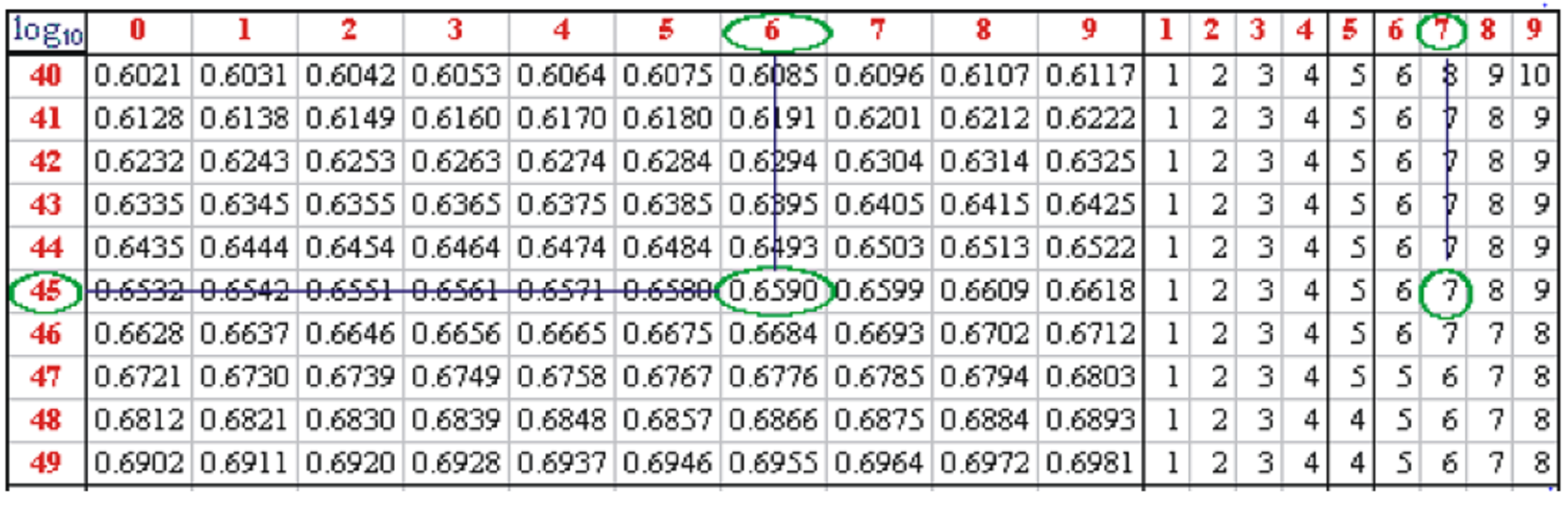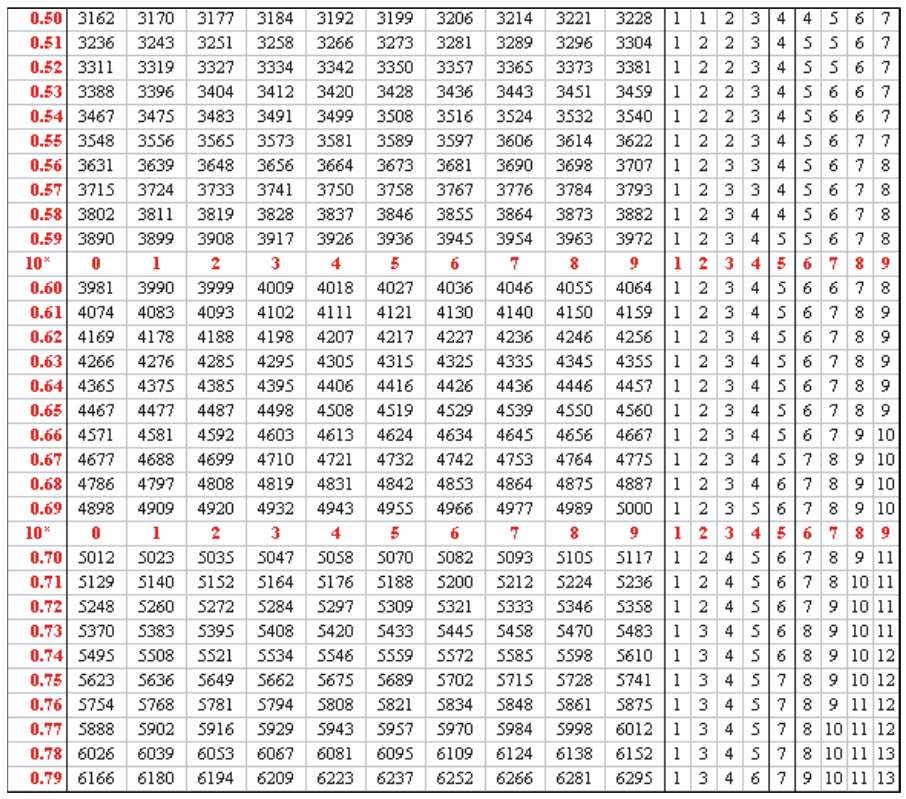# Logs in Calculations

Logs in Calculations

Let us first discuss the general approach for using logs in calculation. Suppose that we have to evaluate the value of N given by

$N = \frac{{a \times b \times {c^{{e_1}}}}}{{x \times {y^{{e_2}}}}}$

One way is to use calculators! But you’ve only your pen and paper and what is called log-tables (we’ll soon discuss these) with you.

Taking the log of both sides to some base B, we have

\begin{align}&{\log _B}N\\& = {\log _B}\left( {\frac{{a \times b \times {c^{{e_1}}}}}{{x \times {y^{{e_2}}}}}} \right)\\& = {\log _B}\left( {a \times b \times {c^{{e_1}}}} \right) - {\log _B}\left( {x \times {y^{{e_2}}}} \right)\\&= \left\{ {{{\log }_B}a + {{\log }_B}b + {{\log }_B}{c^{{e_1}}}} \right\} - \left\{ {{{\log }_B}x + {{\log }_B}{y^{{e_2}}}} \right\}\\& = {\log _B}a + {\log _B}b + {e_1}{\log _B}c - {\log _B}x - {e_2}{\log _B}y\end{align}

Thus, if we have the values of the various logs on the right, we can evaluate the value of logB N. Suppose this comes out to be Z. We then have:

${\log _B}N = Z$

Now we simply take the antilog of both sides, which is nothing but raising both sides to the power B, so that we have

$N = {B^Z}$

Thus, we’ve succeeded in evaluating N using only the operations of additions and subtractions (and simple multiplications). We’ve also taken logs and antilog in the process – but for that we’ve log tables with us.

Before actually doing some calculations, let us understand how to use log tables. The first point is to note is that our default base that we use in 10, i.e., B = 10, so you’ll find log tables generally in base 10. This is because 10 is a very easy base for our minds to grasp, and calculations in base 10 are the easiest (we’ve used base 10 ever since we learnt to count).

Suppose that we have to evaluate the log of 347 to base 10. From now on, this will be written simply as log 347, where the base should be understood to be 10. Note that we can write 347 as

$347\;=\;3.47\;\times\;10^2$

Taking the log of both sides, we have

\begin{align}&\log \,\,347\quad = \log \left( {3.47} \right) + \log \left( {{{10}^2}} \right)\\&\qquad\qquad\; = 2 + \log \left( {3.47} \right)\end{align}

Thus, evaluating log 347 is equivalent to evaluating log 3.47 – the difference is simply of 2. Consider another example:

\begin{align}&6478.25=6.47825\times10^{3\;}\\&\Rightarrow\log\left(6478.25\right)=3+\log\left(6.47825\right)\end{align}

In this manner, taking the log of any number can be reduced to finding the log of a corresponding number between 1 and 10. As another example,

\begin{align}&0.000134{\rm{ }} = {\rm{ }}1.34{\rm{ }} \times {\rm{ }}{10^{--4}}\\ &\Rightarrow \,\,\,\log \left( {0.000134} \right) = \,\,\, - 4 + \log \left( {1.34} \right)\end{align}

The integral part of any log is called its characteristic, while the non-integral part is its mantissa. Note that the mantissa will always lie between 0 and 1. Why? Because the mantissa is the log of some number x between 1 and 10, and if$$1 \le x < 10$$, then $$0 \le \log x < 1$$. Thus, the mantissa lies in [0, 1).

Let us consider some more examples:Thus, the log of any number N will be in the form

$\log N=\left(Characteristic\right)\cdot\left(Mantissa\right)$

such that

$N\;=\;anti\log(Mantissa)\;\times\;10^{Characteristic}$

where antilog(Mantissa) is simply 10(Mantissa)

Note that the Mantissa is always positive (why?), but characteristics will be negative for numbers less than 1.0.

An alert reader should by now have realized that we need log tables only for values between 1 and 10.

Let us use log tables to find log (45.67). We have

\begin{align}&45.67\;=\;4.567\;\times\;10^1\\&\log\;(45.67)\;=\;1\;+\;\log\;(4.567)\end{align}

Thus, we need to find log 4.567. Look up a standard log table. In the left column, locate “45”. If the number that we were looking for were “4500”, the mantissa would have been 0.6532. But now scan the topmost row, and find “60”. Thus, corresponding to “4560”, the mantissa would’ve been 0.6590.

But we actually want the mantissa corresponding to “4567” – thus, add the number from the appropriate column in the right hand set of columns to the mantissa of the first three digits. Thus, the mantissa we are looking for is 0.6590 + 7 = 0.6597.

\begin{align}&\Rightarrow\log\left(4.567\right)=0.6597\\\\&\Rightarrow\log(45.67)=1.6597\end{align}On the other hand, suppose we had been given that the log of N is 1.6597, i.e.,log N  =  1.659

log N  =  1.6597

We need to find N. Now we’ll have to use antilog tables. The approach is the same: have a look at this table:Thus,

$\mathrm{anti}\log\;(1.6597)\;=\;45.67$

Now that you know how to use log and antilog tables, let us perform some calculations using logs.

Example 1: Use logs to evaluate $N = \frac{{647 \cdot 32 \times 0.00000147}}{{8.473 \times 64}}$

Solution: Our approach consists of four steps:

1. convert the expression for N into logs

2. evaluate those logs using a log table

3. thus determine log N

4. calculate antilog of log N

Thus,

\begin{align}&\log N = \left( {\frac{{647 \cdot 32 \times 0.00000147}}{{8.473 \times 64}}} \right)\\\,\,\,\,\,\,\,\,\,\,\,\,\, &= \log \left( {647 \cdot 32} \right) + \log \left( {0.00000147} \right)\\\,\,\,\,\,\,\,\,\,\,\,\,\,\,\,\,\,\,\,\,\,\,\,\,\,\,\,\,\,\,\,\,\,\,\,\,\,\, &- \log \left( {8.473} \right) - \log \left( {64} \right)\end{align}

Now, note that (use log tables):

\begin{align}&647.32=6.4732\times10^2\\&\Rightarrow\log\left(647.32\right)=2+\log\left(6.4732\right)\\&=2+0.8111=2.8111\\&0.00000147=1.47\times10^{-6}\\&\Rightarrow\log\left(0.00000147\right)=-6+\log\left(1.47\right)\\\;\;\;\;\;\;\;\;\;\;\;\;\;\;\;\;\;\;\;\;\;\;\;\;\;\;\;\;\;\;\;\;\;\;\;&=\overline6.1673\\&8.473=8.473\times10^0\\&\Rightarrow\log\left(8.473\right)=0+0.9280\\\;\;\;\;\;\;\;\;\;\;\;\;\;\;\;\;\;\;\;\;\;\;\;&=0.9280\\&64=6.4\times10^1\\&\Rightarrow\log\left(64\right)=1+\log\left(64\right)\\\;\;\;\;\;\;\;\;\;\;\;\;\;\;\;\;\;\;&=1.8062\end{align}

Thus,

\begin{align}&\log N=2.8111+\overline6.1673–0.9280–1.8062\\&\qquad\;=2.8111+\left(–6+0.1673\right)–0.9280–1.8062\\&\qquad\;=–5.7558=–5–0.7558\\&\qquad\;=\left(–5–1\right)+1–0.7558\\&\qquad\;=–6+0.2442\\&\qquad\;=\overline6\cdot2442\end{align}

Note the last couple of steps carefully. Now, we have the characteristic and mantissa of log N.

We thus find antilog (mantissa) = antilog (0.2442) = 1.7547 {from the antilog tables}:

$\Rightarrow \,\,\,N = 1.7547 \times {10^{ - 6}} = 0.00000\,17547$

Verify this using a calculator. Note that N was calculated without any actual multiplication and division – operations much more cumbersome than addition or subtraction.

Example 2: Find the value of $$\sqrt{{0.00000165}}$$

Solution: Let $$N = \sqrt{{0.00000165}}$$

$\begin{array}{l} \Rightarrow \,\,\,\log \,N = \frac{1}{5}\log \left( {0.00000165} \right)\\\\\,\,\,\,\,\,\,\,\,\,\,\,\,\,\,\,\,\,\,\,\, = \frac{1}{5}\left( { - 6 + \log 1.65} \right)\,\,\,{\rm{(why?)}}\end{array}$

Using the log tables, we have:

$\begin{array}{l}\log \,N = \frac{1}{5}\left( { - 6 + .2175} \right) = - 1.2 + 0.0435\\\\\,\,\,\,\,\,\,\,\,\,\,\,\, = - 2 + 0.8435 = \bar 2 \cdot 8435\end{array}$

Thus,

\begin{array}{l}N=anti\log\;\left(0.8435\right)\;10^{-2}\\\;=6.974\;\times\;10^{-2}\;=0.06974\end{array}

Example 3:

How many digits will there be in 87516?

Solution: Let N = 87516. We have:

$\begin{array}{l}\Rightarrow\ log N=16log(875)\\\;\;\;\;\;\;\;\;\;\;\;\;\;\;=16log\{{log(8.75\times10^2)}\}\\\;\;\;\;\;\;\;\;\;\;\;\;\;\;=16(2+log8.75)\\\;\;\;\;\;\;\;\;\;\;\;\;\;\;\;=16\times2.9420\\\;\;\;\;\;\;\;\;\;\;\;\;\;\;\;=47.0721\end{array}$

$\Rightarrow \,\,\,N = \underbrace {{\rm{antilog}}\left( {{\rm{0}}{\rm{.0721}}} \right)}_{\scriptstyle{\rm{Contains \,1\, digit \,before }}\atop\scriptstyle\,\,\,\,\,\,\,\,\,\,\,{\rm{the \,decimal}}}\,\, \times \,\,\underbrace {{\rm{1}}{{\rm{0}}^{{\rm{47}}}}}_{\scriptstyle{\rm{has \,47}}\atop\scriptstyle{\rm{zeroes}}}$

Thus, N will contain 48 digits. Note that we do not need to calculate the antilog of 0.0721 to arrive at this final answer.

Example 4: Evaluate $$\frac{{{{\left( {\frac{{330}}{{49}}} \right)}^4}}}{{\sqrt{{22 \times 70}}}}$$

Solution: If we let N denote this expression, we have

\begin{align} &\log N = 4\log \left( {\frac{{330}}{{49}}} \right) - \frac{1}{3}\log \left( {22 \times 70} \right)\\\,\,\,\,\,\,\,\,\,\,\,\,\, & = 4\log 330 - 4\log 49 - \frac{1}{3}\log 22 - \frac{1}{3}\log 70\end{align}

Noticing that $$330 = 11 \times 3 \times 10,\,\,49\, = {7^2},\,\,22 = 2 \times 11$$ and $$70=7\;\times\;10$$, we have

$\begin{array}{l} \log N = 4\left( {1 + \log 3 + \log 11} \right) - 8\log 7\\ \,\,\,\,\,\,\,\,\,\,\,\,\,\,\,\,\,\,\,\,- \frac{1}{3}\left( {\log 2 + \log 11} \right) - \frac{1}{3}\left( {1 + \log 7} \right)\\ \,\,\,\,\,\,\,\,\,\,\,\,\, = \frac{{11}}{3} - \frac{1}{3}\log 2 + 4\log 3 - \frac{{25}}{3}\log 7 + \frac{{11}}{3}\log 11\\ \,\,\,\,\,\,\,\,\,\,\,\,\, = \frac{{11}}{3} - \frac{1}{3}\left( {0.3010} \right) + 4\left( {0.4771} \right)\\ \,\,\,\,\,\,\,\,\,\,\,\,\,\,\,\,\,\,\,\, - \frac{{25}}{3}\left( {0.8451} \right) + \frac{{11}}{3}\left( {1.0414} \right) \end{array}$

$\begin{array}{l} = 3.6666 - 0.1003 + 1.9084 - 7.0425 + 3.8185\\ = 2.2507\end{array}$

Thus,

$N = {\rm{antilog}}\left( {0.2507} \right) \times {10^2} = 178.1148$

The final result obtained this way may differ somewhat from the actual result due to rounding-off errors along the way.

Example 5:

Solve the following system of equations for x and y :

${2^{x + y}} = {6^y}$

${3^x} = {3.2^{y + 1}}$

Solution: We have

$\begin{array}{l}(x+y)\log2=y\log6\;\;\\\;\;\;\;\;\;\;\;\;\;\;\;\;\;\;\;\;\;\;=y(\log2+\log3)\end{array}$

$$\Rightarrow x\log2=y\log3\;\;\;\;\;\;\;\;\;\;\;\;\;\;\;\;\;\;\;\;\;\;\;\;\;\;\;\;\cdots(1)$$
and, $$x\log 3 = \log 3 + \left( {y + 1} \right)\log 2$$

$\Rightarrow\;\;\;\;\left(x-1\right)\log3=\left(y+1\right)\log2\;\;\;\;\;\;\;\;\;\;\;\;\;\;\dots\left(2\right)$

Using (1) and 2, we have

$x\log 2 = \left( {\frac{{\left( {x - 1} \right)\log 3}}{{\log 2}} - 1} \right)\log 3$

Using log 2 = a, log 3 = b for convenience, we have

$ax = \left( {\frac{{b\left( {x - 1} \right)}}{a} - 1} \right)b$

$\Rightarrow\frac abx=\frac bax-\frac ba-1$

$\Rightarrow\left(\frac ba-\frac ab\right)x=1+\frac ba=\frac{a+b}a$

$\Rightarrow\left(\frac{b^2-a^2}{ab}\right)x=\frac{a+b}a$

$\Rightarrow x = \frac{b}{{b - a}} = \frac{{\log 3}}{{\log 3 - \log 2}} \approx \frac{{ \cdot 4771}}{{ \cdot 1761}} \approx 2.71$

Similarly, $$y \approx 1.71$$.

Example 6: Prove that the following relation is correct for three numbers a, b and c (assume that all the terms are well-defined):

${\log _a}b = \frac{{{{\log }_c}b}}{{{{\log }_c}a}}$

Solution: We suppose the following:

${\log _c}b = x,\,\,\,{\log _c}a = y,\,\,\,{\log _a}b = z$

Thus,

\begin{align}&\left\{ \begin{array}{l}{c^x} = b,\,\,\,{c^y} = a\\{a^z} = b\end{array} \right.\\& \Rightarrow \,\,\,{\left( {{c^y}} \right)^z} = b = {c^x}\\& \Rightarrow \,\,\,{c^{yz}} = {c^x}\,\,\, \Rightarrow \,\,\,yz = x\\ &\Rightarrow \,\,\,z = \frac{y}{x}\,\,\, \Rightarrow \,\,\,{\log _a}b = \frac{{{{\log }_c}b}}{{{{\log }_c}a}}\end{align}

This relation enables us to change bases. For example, suppose that we have to calculate $${\log _{32}}2048$$. We can change the base to 2, as follows:${\log _{32}}2048 = \frac{{{{\log }_2}2048}}{{{{\log }_2}32}} = \frac{{11}}{5} = 2.2$

## What is the use of logs?

• Logs are useful for quantifying the relative change of a value as opposed to its absolute difference.

## How do you do logs on a calculator?

• The "Log" function is available on scientific calculators. It is a key that allows you to work with logarithms.For eg, using the "Log" function on the number 10 would reveal that you have to multiply your base number of 10 by itself one time to equal the number 10.

## How do you find the log and antilog value?

• To compute the antilog of a number y, you must raise the log base b (usually 10, sometimes the constant e) to the power that would generate the number y. Here x is the exponent and y is the antilog value. Consider the equation log(6) = x, its antilog will be 10x = 6.

## What does log mean on a calculator?

• The "Log" function on a graphing or scientific calculator is a key that allows us to work with logarithms. Logarithms are ways to find out what exponents are needed to multiply into a specific number. The number displayed is the exponent for the original number entered.

## What are the logarithm rules?

• There are 7 logarithm rules: Product rule, Quotient rule,Power rule, Zero rule, Identity rule, Log of Exponent Rule and Exponent of Log rule
Exponents and Logarithms
Grade 9 | Questions Set 1
Exponents and Logarithms
Exponents and Logarithms
Grade 9 | Questions Set 2
Exponents and Logarithms
More Important Topics
Numbers
Algebra
Geometry
Measurement
Money
Data
Trigonometry
Calculus
More Important Topics
Numbers
Algebra
Geometry
Measurement
Money
Data
Trigonometry
Calculus